# python中的除法，取整和求模

+关注继续查看

python中的除法，取整和求模http://www.bieryun.com/1102.html1. /是精确除法，//是向下取整除法，%是求模
2. %求模是基于向下取整除法规则的
3. 四舍五入取整round, 向零取整int, 向下和向上取整函数math.floor, math.ceil
4. //和math.floor在CPython中的不同
5. /在python 2 中是向下取整运算
6. C中%是向零取整求模。


1. 一个测试程序和它的输出
2. 取整和求模运算规则
3. python中其他的取整运算
4. //和math.floor在CPython中的不同
5. 运算符/在python 2 和python 3 中的区别
6. 其他讨论(求余和求模)

1. 一个测试程序和它的输出

print('usage of 3 operators /, // and % in python 3.4')
print('1). usage of /')
print ('10/4 = ', 10/4)
print ('-10/4 = ', -10/4)
print ('10/-4 = ', 10/-4)
print ('-10/-4 = ', -10/-4)

print('\n2). usage of //')
print ('10//4 = ', 10//4)
print ('-10//4 = ', -10//4)
print ('10//-4 = ', 10//-4)
print ('-10//-4 = ', -10//-4)

print('\n3). usage of %')
print ('10%4 = ', 10%4)
print ('-10%4 = ', -10%4)
print ('10%-4 = ', 10%-4)
print ('-10%-4 = ', -10%-4)

usage of 3 operators /, // and % in python 3.4
1). usage of /
10/4 =  2.5
-10/4 =  -2.5
10/-4 =  -2.5
-10/-4 =  2.5

2). usage of //
10//4 =  2
-10//4 =  -3
10//-4 =  -3
-10//-4 =  2

3). usage of %
10%4 =  2
-10%4 =  2
10%-4 =  -2
-10%-4 =  -2

2.取整和求模运算规则3.python中其他的取整运算

-10.6//2.1=-6.0
-10.6//2.1=2.00000…

python能够实现基本的四种取整运算：四舍五入，向零取整，向下取整和向上取整

>>>round(3.5)
4
>>>round(-3.5)
-4
>>>round(3.49)
3
>>>round(-3.49)
-3

>>>int(3.99)
3
>>>int (-3.99)
-3

math模块中向下取整函数floor和向上取整函数ceil

>>>from math import floor, ceil
>>>floor(3.99)
3
>>>floor(-3.-1)
-4
>>>ceil(3.01)
4
>>>ceil(-3.99)
-3

4.//和math.floor在CPython中的不同

>>>from math import floor
>>>3//5 == floor(3/5)
True

>>>1//0.05 == floor(1/0.05)
False

What? 不是说好的相同吗，友谊的小船怎么说翻就翻呢？

>>> 1/0.05
20.0
>>> 1//0.05
19.0
>>> floor(1/0.05)
20

PS：Jython下可以得到20.0，而PEP里规定了a // b应该等于round(a/b)，所以似乎这是cpython实现的一个bug？

5.运算符/在python 2 和python 3 中的区别

//和%运算符在2和3版本中一样，但是运算符/不一样，最开始的测试程序中和运算符/有关的部分在python 2.7中的输出结果是这样的：

print ('10/4 = ', 10/4)
print ('-10/4 = ', -10/4)
print ('10/-4 = ', 10/-4)
print ('-10/-4 = ', -10/-4)('1

('10/4 = ', 2)
('-10/4 = ', -3)
('10/-4 = ', -3)
('-10/-4 = ', 2)

6.其他讨论(求余和求模)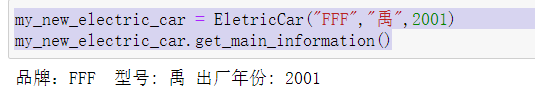0 0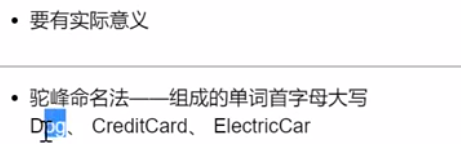0 0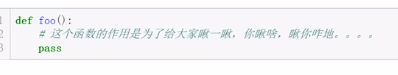0 0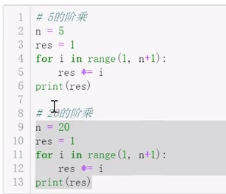0 0Python编程：MySQLdb模块对数据库的基本增删改查操作
Python编程：MySQLdb模块对数据库的基本增删改查操作
0 0Python编程：entry_points将Python模块转变为命令行工具
Python编程：entry_points将Python模块转变为命令行工具
0 0Python编程：SQLAlchemy查询数据没有变化

0 0Python编程：运行目录或zip压缩文件
Python编程：运行目录或zip压缩文件
0 0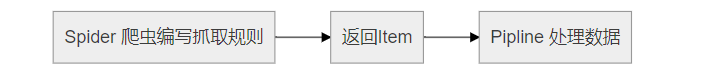Python编程：PyThink数据库交互模块提高爬虫编写速度
Python编程：PyThink数据库交互模块提高爬虫编写速度
0 0Python编程：glob模块进行文件名模式匹配
Python编程：glob模块进行文件名模式匹配
0 0

Python语言基础 - 列表、元组、字典、集合5288020

Python语言基础 - 函数、面向对象、异常处理5452653

Python 科学计算库 NumPy 快速入门4037914

Python语言基础 - 语法入门8939357

Python 脚本进阶12834

【Python入门】Python数据处理编程基础2726

From Python Scikit-Learn to Sc

Python系列直播第一讲——Python中的一切皆对象

Python第五讲——关于爬虫如何做js逆向的思路